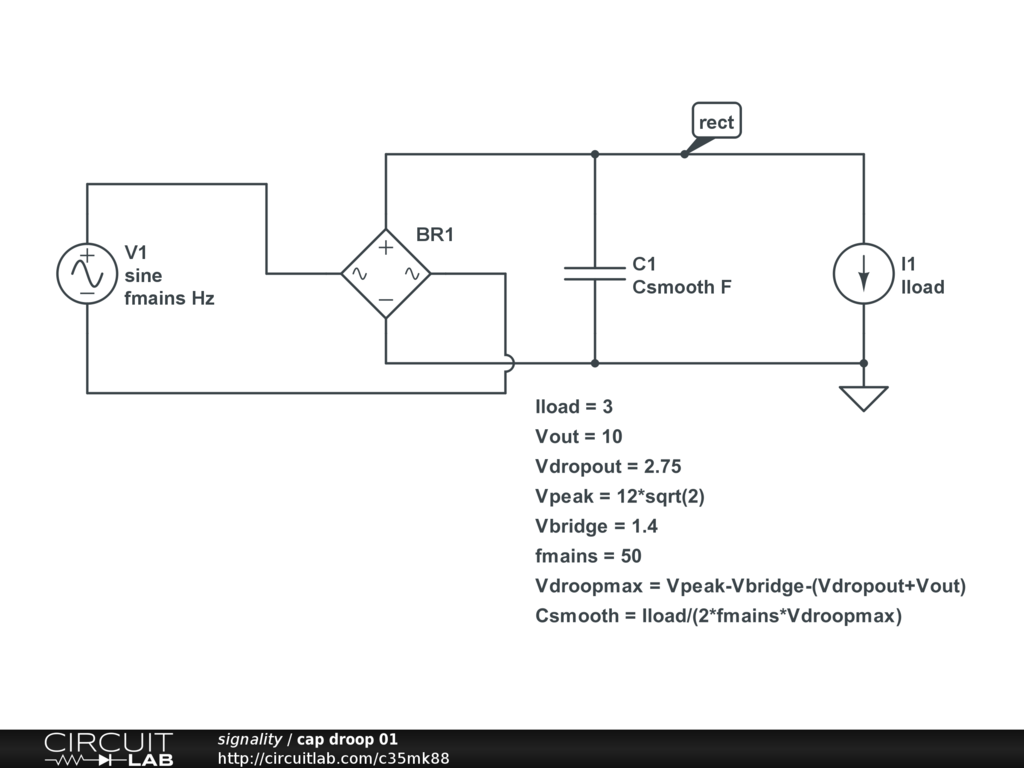## Finding the best ripple voltage.

 I'm looking at the LM350 regulator : https://www.fairchildsemi.com/datasheets/LM/LM350.pdf I'm trying to figure out the correct ripple voltage for the IC. my idea is a power supply that goes up to 10 volts and 3 amps. I found a transformer that steps down from 120 to 12 VAC by Narra November 17, 2014 You need to look at Figure 5. Dropout Voltage, in: https://www.fairchildsemi.com/datasheets/LM/LM350.pdf That tells you the minimum input-output voltage difference that there has to be across the device at various load currents and device temperatures for it to work in regulation. If your transformer is 12V rms then using a full wave bridge recitifer you can expect to get: `Vinmax=(12V*sqrt(2)-1.4V)` peak out of it into the smoothing capacitors allowing for the diode drops when drawing a load current. If you then consider that the input to a linear regulator looks like a constant current sink equal to `Iload` (`Iload` is independent of `Vin` hence it looks like a constant current at the regulator input), then the droop in the capacitor voltage is approximately: `Vdroop=Iload/(2*fmains*Csmooth)` where `fmains` = your local mains frequency `Csmooth` is the total smoothing capacitance. (The formula comes from the charge in a capacitor `Q = C*V=I*t`). Rearranging you can say that the minimum working Vin of the regulator is given by: `Vinmin = Vout + Vdropoutmax` so the max `Vdroop` is given by: `Vdroopmax = Vinmax-Vinmin` Hence `Csmoothmin = Iload/(2*fmains*Vdroopmax)` You also have to work out the worst case for Vinmax at the lowest mains voltage and for Vinmin with the highest dropout voltage with the output voltage at the maximum end of the tolerance. Allow for the maximum load current. Plus allow for capacitor tolerance (and voltage coefficient of capacitance if using ceramic caps with anything poorer than C0G or NP0 dielectric), tolerance of diode voltage drops and transformer tolerances. This can is demonstrated in this parameterised time domain simulation::) by signality November 17, 2014